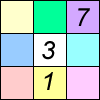Search by Topic

Resources tagged with Working systematically similar to Magical Products:

Filter by: Content type:
Age range:
Challenge level:

There are 127 results

Broad Topics > Using, Applying and Reasoning about Mathematics > Working systematicallyA First Product Sudoku

Age 11 to 14 Challenge Level:

Given the products of adjacent cells, can you complete this Sudoku?How Old Are the Children?

Age 11 to 14 Challenge Level:

A student in a maths class was trying to get some information from her teacher. She was given some clues and then the teacher ended by saying, "Well, how old are they?"Age 7 to 14 Challenge Level:

I added together some of my neighbours' house numbers. Can you explain the patterns I noticed?Product Sudoku

Age 11 to 14 Challenge Level:

The clues for this Sudoku are the product of the numbers in adjacent squares.Multiply the Addition Square

Age 11 to 14 Challenge Level:

If you take a three by three square on a 1-10 addition square and multiply the diagonally opposite numbers together, what is the difference between these products. Why?American Billions

Age 11 to 14 Challenge Level:

Play the divisibility game to create numbers in which the first two digits make a number divisible by 2, the first three digits make a number divisible by 3...Multiples Sudoku

Age 11 to 14 Challenge Level:

Each clue in this Sudoku is the product of the two numbers in adjacent cells.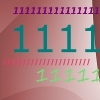Ones Only

Age 11 to 14 Challenge Level:

Find the smallest whole number which, when mutiplied by 7, gives a product consisting entirely of ones.Summing Consecutive Numbers

Age 11 to 14 Challenge Level:

15 = 7 + 8 and 10 = 1 + 2 + 3 + 4. Can you say which numbers can be expressed as the sum of two or more consecutive integers?Factor Lines

Age 7 to 14 Challenge Level:

Arrange the four number cards on the grid, according to the rules, to make a diagonal, vertical or horizontal line.Star Product Sudoku

Age 11 to 16 Challenge Level:

The puzzle can be solved by finding the values of the unknown digits (all indicated by asterisks) in the squares of the $9\times9$ grid.LCM Sudoku

Age 14 to 16 Challenge Level:

Here is a Sudoku with a difference! Use information about lowest common multiples to help you solve it.Ben's Game

Age 11 to 14 Challenge Level:

Ben passed a third of his counters to Jack, Jack passed a quarter of his counters to Emma and Emma passed a fifth of her counters to Ben. After this they all had the same number of counters.Cuboids

Age 11 to 14 Challenge Level:

Find a cuboid (with edges of integer values) that has a surface area of exactly 100 square units. Is there more than one? Can you find them all?Special Numbers

Age 11 to 14 Challenge Level:

My two digit number is special because adding the sum of its digits to the product of its digits gives me my original number. What could my number be?Diagonal Product Sudoku

Age 11 to 16 Challenge Level:

Given the products of diagonally opposite cells - can you complete this Sudoku?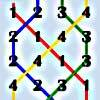Oranges and Lemons, Say the Bells of St Clement's

Age 11 to 14 Challenge Level:

Bellringers have a special way to write down the patterns they ring. Learn about these patterns and draw some of your own.More on Mazes

Age 7 to 14

There is a long tradition of creating mazes throughout history and across the world. This article gives details of mazes you can visit and those that you can tackle on paper.Extra Challenges from Madras

Age 11 to 14 Challenge Level:

A few extra challenges set by some young NRICH members.Peaches Today, Peaches Tomorrow...

Age 11 to 14 Challenge Level:

Whenever a monkey has peaches, he always keeps a fraction of them each day, gives the rest away, and then eats one. How long could he make his peaches last for?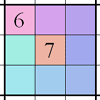Integrated Product Sudoku

Age 11 to 16 Challenge Level:

This Sudoku puzzle can be solved with the help of small clue-numbers on the border lines between pairs of neighbouring squares of the grid.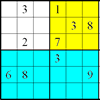Colour Islands Sudoku

Age 11 to 14 Challenge Level:

An extra constraint means this Sudoku requires you to think in diagonals as well as horizontal and vertical lines and boxes of nine.Age 11 to 16 Challenge Level:

The items in the shopping basket add and multiply to give the same amount. What could their prices be?LCM Sudoku II

Age 11 to 18 Challenge Level:

You are given the Lowest Common Multiples of sets of digits. Find the digits and then solve the Sudoku.Magic Potting Sheds

Age 11 to 14 Challenge Level:

Mr McGregor has a magic potting shed. Overnight, the number of plants in it doubles. He'd like to put the same number of plants in each of three gardens, planting one garden each day. Can he do it?Cinema Problem

Age 11 to 14 Challenge Level:

A cinema has 100 seats. Show how it is possible to sell exactly 100 tickets and take exactly £100 if the prices are £10 for adults, 50p for pensioners and 10p for children.More Magic Potting Sheds

Age 11 to 14 Challenge Level:

The number of plants in Mr McGregor's magic potting shed increases overnight. He'd like to put the same number of plants in each of his gardens, planting one garden each day. How can he do it?Number Daisy

Age 11 to 14 Challenge Level:

Can you find six numbers to go in the Daisy from which you can make all the numbers from 1 to a number bigger than 25?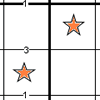Constellation Sudoku

Age 14 to 18 Challenge Level:

Special clue numbers related to the difference between numbers in two adjacent cells and values of the stars in the "constellation" make this a doubly interesting problem.Cayley

Age 11 to 14 Challenge Level:

The letters in the following addition sum represent the digits 1 ... 9. If A=3 and D=2, what number is represented by "CAYLEY"?Two and Two

Age 11 to 14 Challenge Level:

How many solutions can you find to this sum? Each of the different letters stands for a different number.Difference Dynamics

Age 14 to 18 Challenge Level:

Take three whole numbers. The differences between them give you three new numbers. Find the differences between the new numbers and keep repeating this. What happens?Window Frames

Age 5 to 14 Challenge Level:

This task encourages you to investigate the number of edging pieces and panes in different sized windows.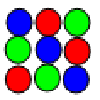Latin Squares

Age 11 to 18

A Latin square of order n is an array of n symbols in which each symbol occurs exactly once in each row and exactly once in each column.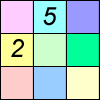Difference Sudoku

Age 14 to 16 Challenge Level:

Use the differences to find the solution to this Sudoku.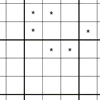Age 11 to 14 Challenge Level:

You need to find the values of the stars before you can apply normal Sudoku rules.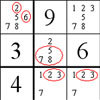The Naked Pair in Sudoku

Age 7 to 16

A particular technique for solving Sudoku puzzles, known as "naked pair", is explained in this easy-to-read article.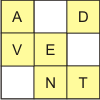Age 11 to 14 Challenge Level:

Rather than using the numbers 1-9, this sudoku uses the nine different letters used to make the words "Advent Calendar".A Long Time at the Till

Age 14 to 18 Challenge Level:

Try to solve this very difficult problem and then study our two suggested solutions. How would you use your knowledge to try to solve variants on the original problem?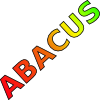Counting on Letters

Age 11 to 14 Challenge Level:

The letters of the word ABACUS have been arranged in the shape of a triangle. How many different ways can you find to read the word ABACUS from this triangular pattern?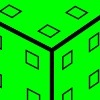Inky Cube

Age 7 to 14 Challenge Level:

This cube has ink on each face which leaves marks on paper as it is rolled. Can you work out what is on each face and the route it has taken?Pair Sums

Age 11 to 14 Challenge Level:

Five numbers added together in pairs produce: 0, 2, 4, 4, 6, 8, 9, 11, 13, 15 What are the five numbers?Age 11 to 14 Challenge Level:

How many different symmetrical shapes can you make by shading triangles or squares?Sticky Numbers

Age 11 to 14 Challenge Level:

Can you arrange the numbers 1 to 17 in a row so that each adjacent pair adds up to a square number?Number Sandwiches

Age 7 to 14 Challenge Level:

Can you arrange the digits 1, 1, 2, 2, 3 and 3 to make a Number Sandwich?9 Weights

Age 11 to 14 Challenge Level:

You have been given nine weights, one of which is slightly heavier than the rest. Can you work out which weight is heavier in just two weighings of the balance?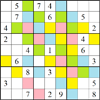Rainstorm Sudoku

Age 14 to 16 Challenge Level:

Use the clues about the shaded areas to help solve this sudokuWeights

Age 11 to 14 Challenge Level:

Different combinations of the weights available allow you to make different totals. Which totals can you make?An Introduction to Magic Squares

Age 7 to 16

Find out about Magic Squares in this article written for students. Why are they magic?!